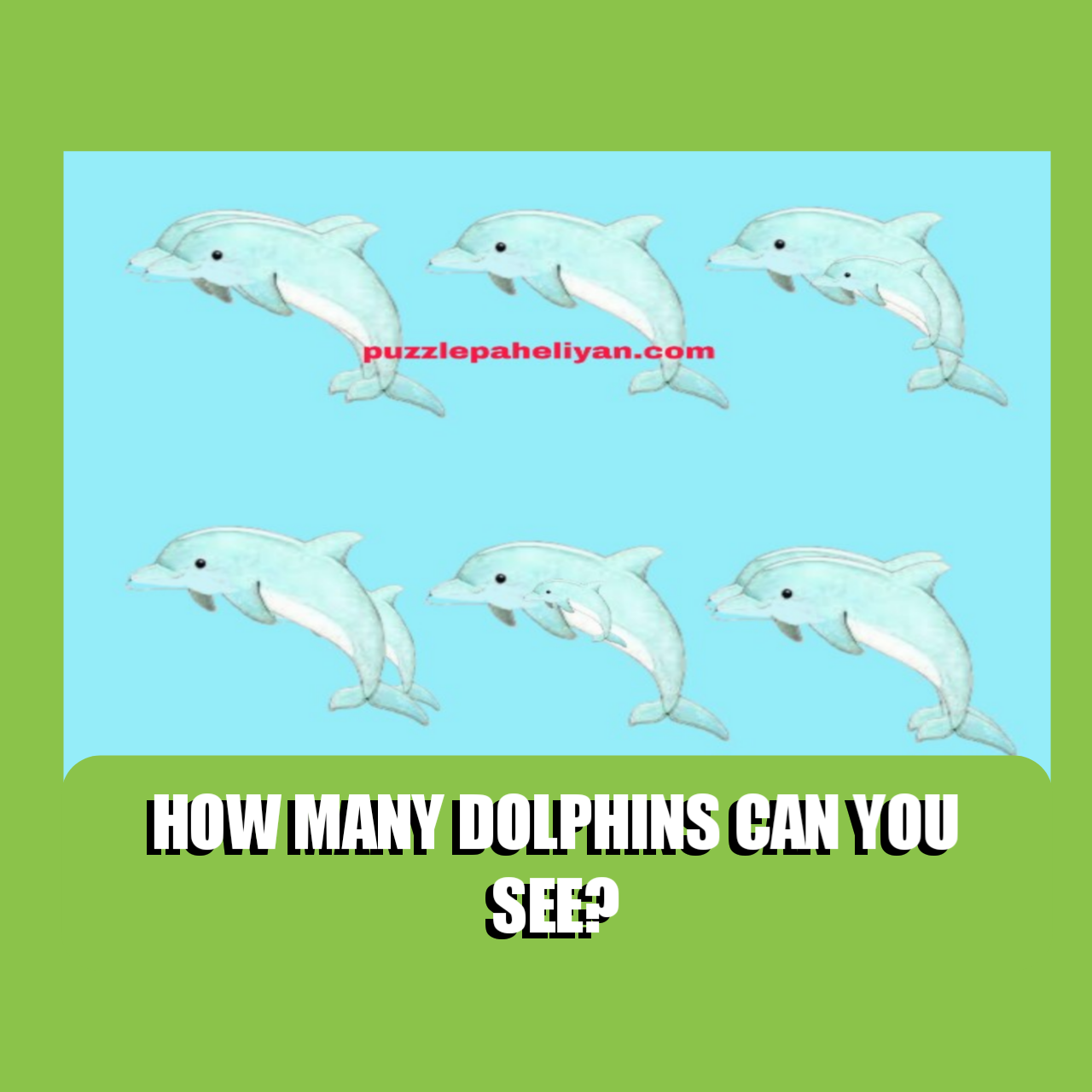0

# Answer to dolphins math picture PuzzleAnswer to dolphins math picture Puzzle

This dolphin math picture Puzzle is one amongst other Math puzzles like the  " How many eggs can you see math Puzzle" that's trending online. The puzzle is aimed to test your focus and attention. Many people have tried answering it on Facebook but failed. Today I will show you the correct answer.

## Dolphins math picture Puzzle answer

The correct answer to the "how many dolphins can you see in the math picture Puzzle" is 11. You need to pay attention to the bigger dolphins because some smaller ones are hanging on bigger ones. Details of the answer below.

Be sure to share the picture Puzzle with friends and test them while catching fun.

The simple way to get the correct answer is to count carefully. I will start from the first line, second line and add up to get the final answer.

Counting from Left to right in the first line,

They're two dolphins at the beginning, one in the middle and two at the end.

Total dolphins in line one = 5

In the second line, they're two dolphins at the beginning, two in the middle and two at the end.

Total Dolphins in line two = 6

Hence total number of Dolphins on the picture math puzzle = 5+6 = 11.

Do you have a different answer?

Share with us